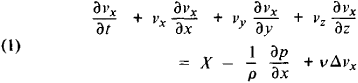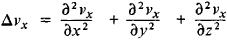# Navier-Stokes Equations

(redirected from Navier Stokes Equations)

## Navier-Stokes equations

[nä′vyā ′stōks i‚kwā·zhənz]
(fluid mechanics)
The equations of motion for a viscous fluid which may be written d V/ dt = -(1/ρ)∇ p + F + ν∇2V + (⅓)ν∇(∇·V), where p is the pressure, ρ the density, F the total external force per unit mass, V the fluid velocity, and ν the kinematic viscosity; for an incompressible fluid, the term in ∇·V (divergence) vanishes, and the effects of viscosity then play a role analogous to that of temperature in thermal conduction and to that of density in simple diffusion.
McGraw-Hill Dictionary of Scientific & Technical Terms, 6E, Copyright © 2003 by The McGraw-Hill Companies, Inc.
The following article is from The Great Soviet Encyclopedia (1979). It might be outdated or ideologically biased.

## Navier-Stokes Equations

the differential equations that describe the motion of a viscous fluid. These equations are named after L. Navier and G. Stokes. For an incompressible (density ρ = constant) and unheated (temperature T = constant) fluid, the Navier-Stokes equations projected on the axes of a rectangular Cartesian coordinate system (a system of three equations) have the formHere t is the time; x, y, and z are the coordinates of a particle of fluid; vx, vy, and vz are the projections of the velocity of the particle; X, Y, and Z are the projections of the body force; ρ is the pressure; ν = μ/ρ is the kinematic viscosity coefficient (where μ is the dynamic viscosity coefficient), andTwo other equations are obtained by replacing x with y, y with z, and z with x.

The Navier-Stokes equations are used to determine vx, vy vz., and ρ as functions of x, y, z, and t. In order to close the system, we add to equations (1) a continuity equation, which for an incompressible fluid has the formIn order to integrate equations (1) and (2), we must be given the initial conditions (if the motion is not steady state) and the boundary conditions, which for a viscous fluid are the conditions of adhesion to rigid walls. In the general case of the motion of a compressible and heated fluid, the Navier-Stokes equations also take into account the variability of ρ and the temperature dependence of μ, changing the form of the equations. In this case, the equation of energy balance and the Clapeyron equation are also used.

The Navier-Stokes equations are used in the study of the motions of real liquids and gases; in most such specific problems, only various approximate solutions are sought.

S. M. TARG

The Great Soviet Encyclopedia, 3rd Edition (1970-1979). © 2010 The Gale Group, Inc. All rights reserved.
References in periodicals archive ?
Models use the Reynolds Averaged Navier Stokes equations (and sometimes the filtered Navier Stokes equations) to calculate the blood flow using a computer model.
Mattingly and Sinai  attempted to show that smooth solutions to 3D Navier Stokes equations exist for all initial conditions u(x, 0) = [u.sup.0](x) [member of] [C.sup.[infinity]] by dealing with an equivalent form of the Navier-Stokes equations for periodic boundary conditions:
(2002) studied the forces acting on cuttings layers based on continuity and Navier Stokes equations. They have analyzed the parameters of annular velocity, pressure gradient and fluid rheology.
Mihail, Numerical solution of the steady incompressible Navier Stokes equations for the flow past a sphere by a multigrid defect correction technique, Internal J.
Exact solution of the Navier Stokes equations for the fully developed, pulsating flow in a rectangular Duct with a constant cross-sectional velocity.
RIVIERE, A discontinuous subgrid eddy viscosity method for the time-dependent Navier Stokes equations, SIAM J.

Site: Follow: Share:
Open / Close